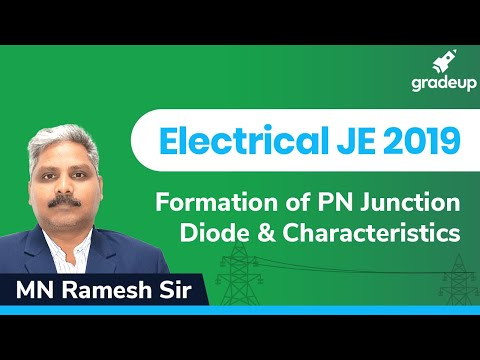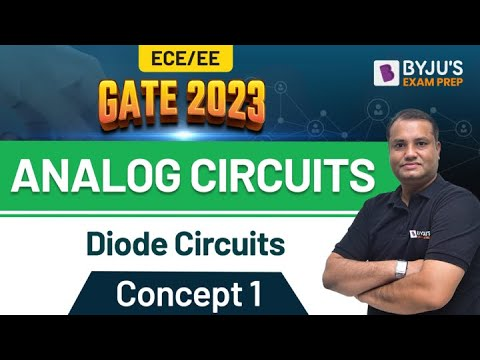Checkout JEE MAINS 2022 Question Paper Analysis : Checkout JEE MAINS 2022 Question Paper Analysis :

# Bridge Rectifier Questions

## What is a Bridge Rectifier?

The circuits that convert AC (Alternating Current) into DC (Direct Current) using diodes that are organised in a bridge circuit configuration are known as bridge rectifiers. These rectifiers generally consist of four or more diodes, and irrespective of the polarity at the input, the wave generated at the output will be of the same polarity.

The bridge rectifier is generally a single-phase type rectifier, though it can also be prolonged to a three-phase rectifier. Both the mentioned rectifiers can be additionally classified into half controlled, uncontrolled and full controlled bridge rectifiers. Since we cannot control the biassing of the diode, the circuit is said to be uncontrolled, but if a thyristor is used to replace all four diodes, its biassing can be controlled through its gate signal by controlling its firing angle. This will result in a rectifier that can be controlled fully. In a bridge rectifier that is half-controlled, half of the circuit contains thyristors, and the other half includes diodes.

## Applications of a Bridge Rectifier

• Bridge Rectifier is used for supplying polarised and stable DC voltage in welding.
• To maintain a stable supply of power, bridge rectifiers are used in power supplies.
• Bridge rectifiers are generally used in battery chargers.
• Bridge rectifiers are used in wind turbines.
• To detect the amplitude of modulating signals, bridge rectifiers are widely used.
• The Bridge rectifier is used for converting the high AC to low DC voltage.

## Important Questions on Bridge Rectifier

1) DC average current of a bridge full wave rectifier is _____.

a) 20Im

b) Im

c) Im/4

d) 1.4Im

Correct Option: (b)

Explanation: Since only one half of the input is involved in the output of the half wave rectifier, Im is the average DC current of half wave rectifier. And also, half of the area of the one-half cycle of a sine wave with a peak is the average value Im.

2) _____ is the ripple factor of bridge full wave rectifiers.

a) 1.111

b) 1.222

c) 0.482

d) 1.321

Correct Option: (c)

Explanation: The ripples or AC content in the output is measured by the ripple factor. For a full wave bridge rectifier, it is 0.482, since it can be calculated by dividing AC rms output with DC output.

3) What will be the ripple frequency of the bridge full wave rectifier, if the input frequency is 50Hz?

a) 500Hz

b) 200Hz

c) 50Hz

d) 100Hz

Correct Option: (d)

Explanation: Since the one-half cycle is repeated in the output of the bridge rectifier, the frequency will be twice as that of the input frequency, so f=100Hz.

4) For a bridge full wave rectifier, the transformer utilisation factor is ________.

a) 0.823

b) 0.812

c) 0.593

d) 0.825

Correct Option: (b)

Explanation: The ratio of AC power delivered to load to the DC power rating is known as the transformer utilisation factor. This factor shows the efficiency of transformer usage by the rectifier; it is equal to 0.693 for a bridge full wave rectifier.

5) Efficiency of bridge full wave rectifiers is _____.

a) 81.2%

b) 55%

c) 42.6%

d) 46.33%

Correct Option: (a)

Explanation: For bridge full wave rectifiers, it’s 81.2%, since the efficiency of a rectifier to convert AC to DC is known as the efficiency of a rectifier, and it is attained by taking the ratio of DC power output to maximum AC power delivered to load.

6) How many diodes are used in a full wave bridge rectifier?

a) 9

b) 1

c) 3

d) 4

Correct Option: (d)

Explanation: The model of Wein Bridge and a rectifier is the same. The Wein Bridge needs four resistors; similarly, the bridge requires four diodes for the rectifier.

7) Which among the following is not a type of rectifier?

a) Peak Rectifier

b) Bridge Rectifier

c) Precision Half-wave Rectifier

d) None of the above

Correct Option: (d)

Explanation: All of the above-mentioned rectifiers are different types of a rectifier.

8) The peak inverse voltage of the rectifier for a full wave or half wave rectifier is always _____.

a) More than the input voltage

b) Lesser than the input voltage

c) Equal to the input voltage

d) None of the above

Correct Option: (b)

Explanation: Due to the presence of diodes, the peak input voltage is lesser than the input voltage because a single diode decreases the output voltage by about 0.7V.

9) For _____, a bridge rectifier is a good alternative.

a) A full wave rectifier

b) A peak rectifier

c) A half wave rectifier

d) None of the above

Correct Option: (a)

Explanation: For a full wave rectifier, a bridge rectifier is a better alternative

10) For a bridge rectifier, which among the following statements is true?

a) When compared to an identical centre tapped rectifier, the peak inverse voltage for the bridge rectifier is low.

b) As compared to the identical bridge rectifier, the output voltage for the centre tapped rectifier is low.

c) In a centre tapped rectifier, a transistor of a higher number of coils is required than the identical bridge rectifier

d) All of the above

Correct Option: (d)

Explanation: For a bridge rectifier, all the above-mentioned statements are true.

### Practice Questions

1) What is a full-wave rectifier?

2) What is a half-wave rectifier?

3) What is the ripple factor?

4) Define bridge rectifier.

5) What is the maximum efficiency of a bridge rectifier?

 Related Articles

## Suggested VideosStay tuned to BYJU’S and Fall in Love with Learning!